Meteorological, 2D{1,1,2,1,1} dataset¶

The following dataset is obtained from NOAA/NCEP Global Forecast System (GFS) Atmospheric Model and subsequently converted to the CSD model file-format. The dataset consists of two spatial dimensions describing the geographical coordinates of the earth surface and five dependent variables with 1) surface temperature, 2) air temperature at 2 m, 3) relative humidity, 4) air pressure at sea level as the four scalar quantity_type dependent variables, and 5) wind velocity as the two-component vector, quantity_type dependent variable.

Let’s import the csdmpy module and load this dataset.

import csdmpy as cp

filename = "https://osu.box.com/shared/static/6uhrtdxfisl4a14x9pndyze2mv414zyg.csdf"

The tuple of dimension and dependent variable objects from multi_dataset instance are

The dataset contains two dimension objects representing the longitude and latitude of the earth’s surface. The labels along thee respective dimensions are

print(x.label)

Out:

longitude
print(x.label)

Out:

latitude

There are a total of five dependent variables stored in this dataset. The first dependent variable is the surface air temperature. The data structure of this dependent variable is

print(y.data_structure)

Out:

{
"type": "internal",
"description": "The label 'tmpsfc' is the standard attribute name for 'surface air temperature'.",
"name": "Surface temperature",
"unit": "K",
"quantity_name": "temperature",
"numeric_type": "float64",
"quantity_type": "scalar",
"component_labels": [
"tmpsfc - surface air temperature"
],
"components": [
[
"292.8152160644531, 293.0152282714844, ..., 301.8152160644531, 303.8152160644531"
]
]
}

If you have followed all previous examples, the above data structure should be self-explanatory.

We will use the following snippet to plot the dependent variables of scalar quantity_type.

import numpy as np
import matplotlib.pyplot as plt
from mpl_toolkits.axes_grid1 import make_axes_locatable

def plot_scalar(yx):
fig, ax = plt.subplots(1, 1, figsize=(6, 3))

# Set the extents of the image plot.
extent = [
x.coordinates.value,
x.coordinates[-1].value,
x.coordinates.value,
x.coordinates[-1].value,
]

im = ax.imshow(yx.components, origin="lower", extent=extent, cmap="coolwarm")

divider = make_axes_locatable(ax)
cbar = fig.colorbar(im, cax)
cbar.ax.set_ylabel(yx.axis_label)

# Set up the axes label and figure title.
ax.set_xlabel(x.axis_label)
ax.set_ylabel(x.axis_label)
ax.set_title(yx.name)

# Set up the grid lines.
ax.grid(color="k", linestyle="--", linewidth=0.5)

plt.tight_layout()
plt.show()

Now to plot the data from the dependent variable.

plot_scalar(y)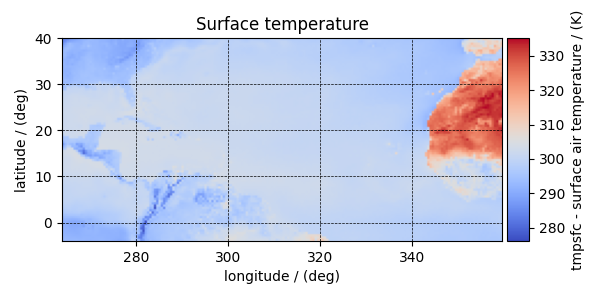Similarly, other dependent variables with their respective plots are

print(y.name)

Out:

Air temperature at 2m
plot_scalar(y)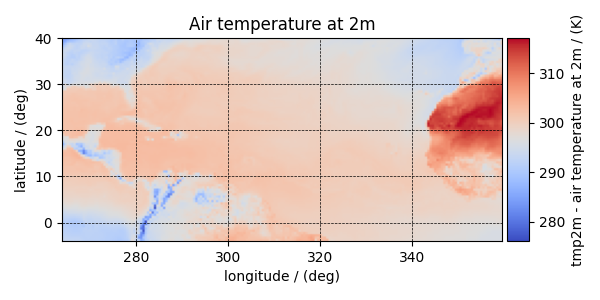print(y.name)

Out:

Relative humidity
plot_scalar(y)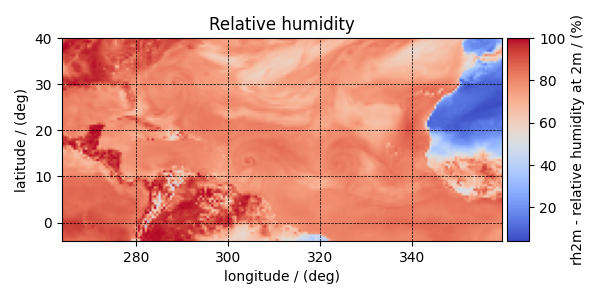print(y.name)

Out:

Air pressure at sea level
plot_scalar(y)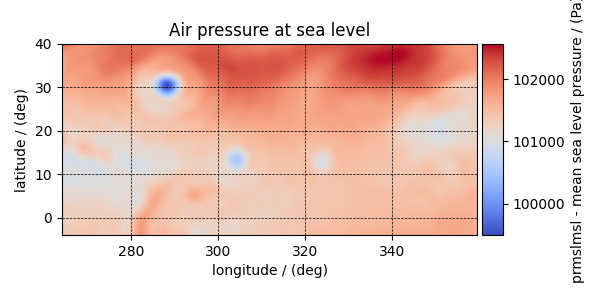Notice, we skipped the dependent variable at index two. The reason is that this particular dependent variable is a vector dataset,

print(y.quantity_type)

Out:

vector_2
print(y.name)

Out:

Wind velocity

which represents the wind velocity, and requires a vector visualization routine. To visualize the vector data, we use the matplotlib quiver plot.

def plot_vector(yx):
fig, ax = plt.subplots(1, 1, figsize=(6, 3))
magnitude = np.sqrt(yx.components ** 2 + yx.components ** 2)

cf = ax.quiver(
x.coordinates,
x.coordinates,
yx.components,
yx.components,
magnitude,
pivot="middle",
cmap="inferno",
)
divider = make_axes_locatable(ax)
cbar = fig.colorbar(cf, cax)
cbar.ax.set_ylabel(yx.name + " / " + str(yx.unit))

ax.set_xlim([x.coordinates.value, x.coordinates[-1].value])
ax.set_ylim([x.coordinates.value, x.coordinates[-1].value])

# Set axes labels and figure title.
ax.set_xlabel(x.axis_label)
ax.set_ylabel(x.axis_label)
ax.set_title(yx.name)

# Set grid lines.
ax.grid(color="gray", linestyle="--", linewidth=0.5)

plt.tight_layout()
plt.show()
plot_vector(y)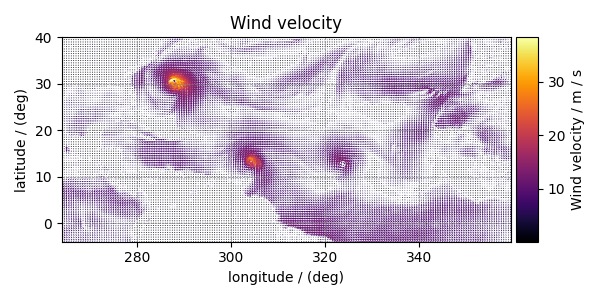Total running time of the script: ( 0 minutes 2.600 seconds)

Gallery generated by Sphinx-Gallery